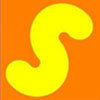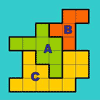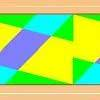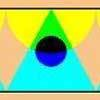# Search by Topic

#### Resources tagged with Dynamic geometry similar to Quad in Quad:

Filter by: Content type:
Age range:
Challenge level:

### There are 20 results

Broad Topics > Information and Communications Technology > Dynamic geometry##### Age 14 to 16 Challenge Level:

Join the midpoints of a quadrilateral to get a new quadrilateral. What is special about it?##### Age 14 to 16 Challenge Level:

The points P, Q, R and S are the midpoints of the edges of a non-convex quadrilateral.What do you notice about the quadrilateral PQRS and its area?### Three Balls

##### Age 14 to 16 Challenge Level:

A circle has centre O and angle POR = angle QOR. Construct tangents at P and Q meeting at T. Draw a circle with diameter OT. Do P and Q lie inside, or on, or outside this circle?### Polycircles

##### Age 14 to 16 Challenge Level:

Show that for any triangle it is always possible to construct 3 touching circles with centres at the vertices. Is it possible to construct touching circles centred at the vertices of any polygon?### Secants Interactivity

##### Age 14 to 16 Challenge Level:

Move the ends of the lines at points B and D around the circle and find the relationship between the length of the line segments PA, PB, PC, and PD. The length of each of the line segments is. . . .### Isosceles

##### Age 11 to 14 Challenge Level:

Prove that a triangle with sides of length 5, 5 and 6 has the same area as a triangle with sides of length 5, 5 and 8. Find other pairs of non-congruent isosceles triangles which have equal areas.##### Age 14 to 16 Challenge Level:

Four rods are hinged at their ends to form a convex quadrilateral. Investigate the different shapes that the quadrilateral can take. Be patient this problem may be slow to load.### Trapezium Four

##### Age 14 to 16 Challenge Level:

The diagonals of a trapezium divide it into four parts. Can you create a trapezium where three of those parts are equal in area?### The Medieval Octagon

##### Age 14 to 16 Challenge Level:

Medieval stonemasons used a method to construct octagons using ruler and compasses... Is the octagon regular? Proof please.### Triangle Incircle Iteration

##### Age 14 to 16 Challenge Level:

Keep constructing triangles in the incircle of the previous triangle. What happens?### Two Shapes & Printer Ink

##### Age 14 to 16 Challenge Level:

If I print this page which shape will require the more yellow ink?### Napoleon's Theorem

##### Age 14 to 18 Challenge Level:

Triangle ABC has equilateral triangles drawn on its edges. Points P, Q and R are the centres of the equilateral triangles. What can you prove about the triangle PQR?### The Rescaled Map

##### Age 14 to 16 Challenge Level:

We use statistics to give ourselves an informed view on a subject of interest. This problem explores how to scale countries on a map to represent characteristics other than land area.### The Line and Its Strange Pair

##### Age 14 to 16 Challenge Level:

In the diagram the point P' can move to different places along the dotted line. Each position P' takes will fix a corresponding position for P. If P' moves along a straight line what does P do ?### Eight Ratios

##### Age 14 to 16 Challenge Level:

Two perpendicular lines lie across each other and the end points are joined to form a quadrilateral. Eight ratios are defined, three are given but five need to be found.### Mapping the Wandering Circle

##### Age 14 to 16 Challenge Level:

In the diagram the point P can move to different places around the dotted circle. Each position P takes will fix a corresponding position for P'. As P moves around on that circle what will P' do?### Rotations Are Not Single Round Here

##### Age 14 to 16 Challenge Level:

I noticed this about streamers that have rotation symmetry : if there was one centre of rotation there always seems to be a second centre that also worked. Can you find a design that has only. . . .### A Roll of Patterned Paper

##### Age 14 to 16 Challenge Level:

A design is repeated endlessly along a line - rather like a stream of paper coming off a roll. Make a strip that matches itself after rotation, or after reflection### One Reflection Implies Another

##### Age 14 to 16 Challenge Level:

When a strip has vertical symmetry there always seems to be a second place where a mirror line could go. Perhaps you can find a design that has only one mirror line across it. Or, if you thought that. . . .### Points in Pairs

##### Age 14 to 16 Challenge Level:

In the diagram the radius length is 10 units, OP is 8 units and OQ is 6 units. If the distance PQ is 5 units what is the distance P'Q' ?